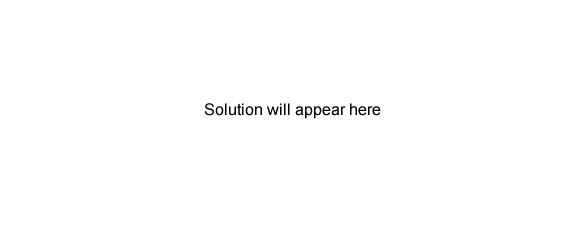Volumetric analysis exercises (2007 VCE) Sulfur dioxide gas is commonly used as a preservative in wine. An important source of SO2 is solid sodium metabisulfite (Na2S2O5; molar mass 190 g/mol). Na2S2O5 reacts readily with acid as follows. Na2S2O5(s) + 2HCl(aq) → 2NaCl(aq) + H2O(l) + 2SO2(g) Calculate the volume, in litres, of SO2 produced at 1.00 atm pressure and 15.0oC when 250 g of Na2S2O5 reacts with excess acid. SolutionThe concentration of an aqueous solution of SO2 (solution A) is to be determined using its reaction with an aqueous solution of triiodide ions (I3). The relevant half reactions are SO2(aq) + 2H2O(l) → 4H+(aq) + SO4-2(aq) + 2e I3 -(aq) + 2e → 3I-(aq) 50.0 mL of a 0.0125 M solution of I3 - is added to 50.0 mL of solution A, providing excess of I3 -. The final 100.0 mL solution is called solution B. Write an overall balanced chemical equation for the reaction that occurs, identifying the substance that is the reductant. Solution Calculate the amount, in mol, of I3 - added to solution A. Solution The excess I3 - remaining in the solution is determined by titration with a standard solution of sodium thiosulfate (Na2S2O3). The equation for the reaction is S2O3-2(aq) + I3 - (aq) + H2O(l) → 3I-(aq) + S2O4-2(aq) + 2H+(aq) 14.70 mL of a 0.00850 M solution of Na2S2O3 reacts exactly with all the I3 - remaining in solution B. Calculate the original concentration of SO2 in solution A. Solution